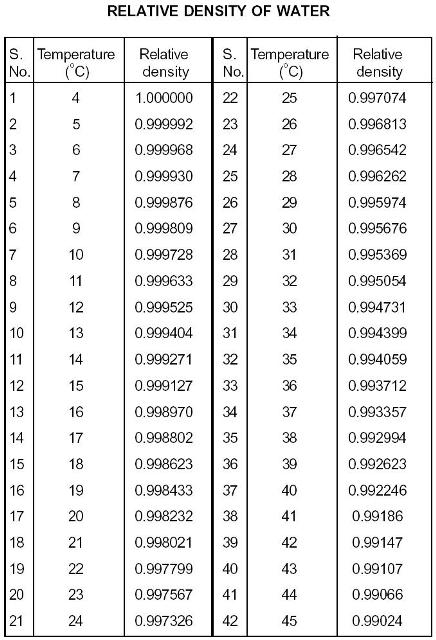Search

# Relative Density Of Water

Posted in Civil Engineering Tests |Email This Post |

The relative density of water is shown in the table below.Also refer to the relative density of soil for more inforamtion on civil engineering tests.

The density varies with temperature and we take the value equal to 1 at a standard temperature of 4oC. The value of density of water changes as the temperature increases and this can be taken directly from the table in various tests like

The Specific Gravity Of Soil Test and so on.More Entries :
• ericj April 11, 2009 at 1:20 pm

what is the relative density of kerosine

• aloysius June 19, 2010 at 2:31 pm

what is the relative density of water and kerosene?

• bejoy September 15, 2011 at 7:49 pm

what is the relative density of water and kerosene?

• smith January 23, 2012 at 9:47 am

wass d reltive density of water n petrl

• edna August 20, 2012 at 3:45 am

Amixture of two liquids of equal volume is made, the one has a relative density of 0.716 and the other 1, 000.498 . What will the weight of 2,964.931.liter

• edna August 20, 2012 at 3:50 am

relation between relative density and weight